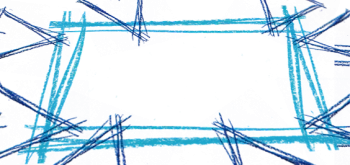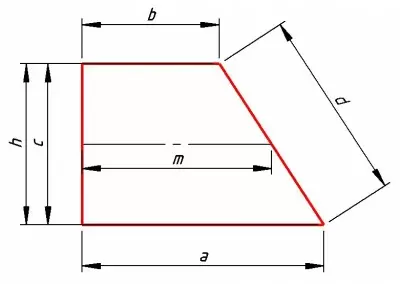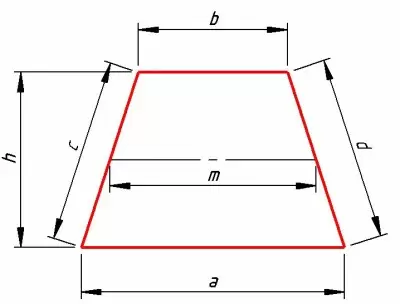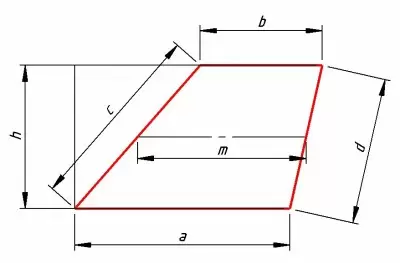# Trapezoid: How to Find Its Surface Area and PerimeterA trapezoid is an irregular polygon made up of four edges and four vertices, making it a convex quadrilateral. It is characterized by having a pair of parallel sides called bases.

The median line is a segment that connects the midpoints of the edges. The height of the trapezoid is the distance between the top base and the bottom base.

A particular case is a rectangle: in this case, it is a trapezoid in which all its internal angles are right. Hence, it has straight sides, and the length of both trapezoid bases are equal.

## How to Find the Surface Area of ​​a Trapezoid

The formula to find the area of ​​a trapezoid that can be solved knowing the length of the two parallel bases "a" and "b" and the height "h" is as follows:Therefore, the area is half the sum of the length of the bases times the height. This area equals the sum of the surface area of ​​a rectangle formed by the short base and the height plus the sum of the surface area of ​​the remaining triangles.

We can also calculate the area if we know the length of all the sides of this type of quadrilateral. In this case, we use the following formula:In the equation above, “a” and “c” are the figure bases, and “b” and “d” correspond to the sides.

## Formula to Find the Perimeter of a Trapezoid

The perimeter of a trapezoid is the sum of the length of the four sides of the figure. Therefore, the formula is as follows:

P=a+b+c+d.

However, there is a particular case which is the isosceles trapezoid. In this type of quadrilateral, the oblique sides are the same length. Therefore, its perimeter is calculated with the add of the length of the top and bottom bases plus one side by two, as shown in the following formula:

P=a+b+2c

## What Are the Three Types of a Trapezoid?

Below we present the three types and images of trapezoids.

### 1. Rectangular Trapezoid

This type of irregular polygon has a side perpendicular to the bases. Therefore, it has two right internal angles, and the height of one side is equal to the height.### 2. Isosceles Trapezoid

This quadrilateral has two sides of the same length, but they are not parallel. Therefore, the pair of opposite sides, “c” and “d,” have equal sizes in the figure.It means that:

• It is a symmetrical flat figure.

• The sum of its opposite interior angles is 180 degrees.

• Two of the interior angles are obtuse, and two are acute.

• The two diagonals are identical.

### 3. Scalene Trapezius

When a trapezoid is neither right nor isosceles, it is classified as scalene.• All interior angles are different.

• All sides of the trapezoid (abcd) have different lengths, as shown in the picture.

Author:

Published: March 31, 2022
Last review: March 31, 2022